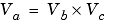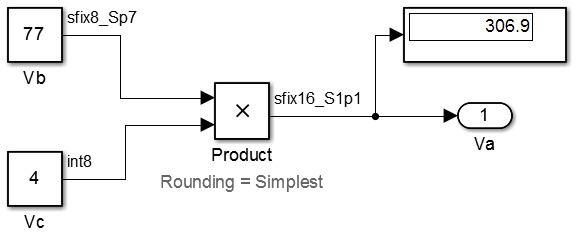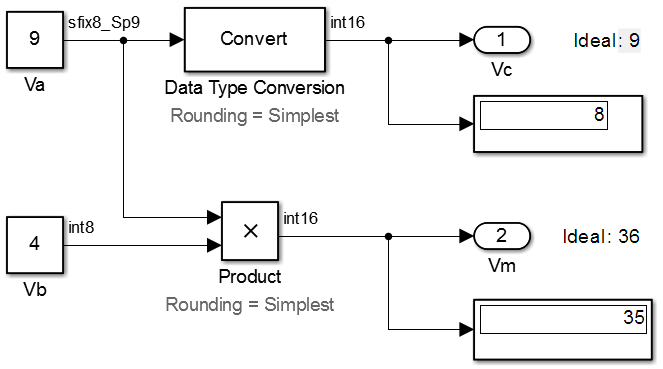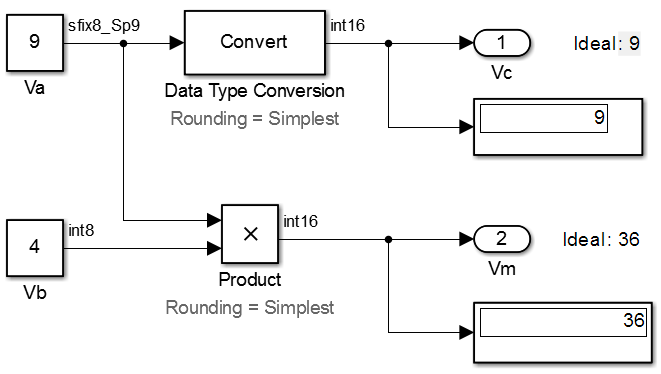## Net Slope Computation

### Handle Net Slope Computation

The Fixed-Point Designer™ software provides an optimization parameter, Use division for fixed-point net slope computation, that controls how the software handles net slope computation. To learn how to enable this optimization, see Use Integer Division to Handle Net Slope Computation.

When a change of fixed-point slope is not a power of two, net slope computation is necessary. Normally, net slope computation is implemented using an integer multiplication followed by shifts. Under certain conditions, net slope computation can be implemented using integer division or a rational approximation of the net slope. One of the conditions is that the net slope can be accurately represented as a rational fraction or as the reciprocal of an integer. Under this condition, the division implementation gives more accurate numerical behavior. Depending on your compiler and embedded hardware, a division implementation might be more desirable than the multiplication and shifts implementation. The generated code for the rational approximation and/or integer division implementation might require less ROM or improve model execution time.

#### When to Use Division for Fixed-Point Net Slope Computation

This optimization works if:

• The net slope can be approximated with a fraction or is the reciprocal of an integer.

• Division is more efficient than multiplication followed by shifts on the target hardware.

Note

The Fixed-Point Designer software is not aware of the target hardware. Before selecting this option, verify that division is more efficient than multiplication followed by shifts on your target hardware.

#### When Not to Use Division to Handle Net Slope Computation

This optimization does not work if:

• The software cannot perform the division using the production target `long` data type and therefore must use multiword operations.

Using multiword division does not produce code suitable for embedded targets. Therefore, do not use division to handle net slope computation in models that use multiword operations. If your model contains blocks that use multiword operations, change the word length of these blocks to avoid these operations.

• Net slope is a power of 2 or a rational approximation of the net slope contains division by a power of 2.

Binary-point-only scaling, where the net slope is a power of 2, involves moving the binary point within the fixed-point word. This scaling mode already minimizes the number of processor arithmetic operations.

### Use Division to Handle Net Slope Computation

To enable this optimization:

1. In the Configuration Parameters dialog box, on the Math and Data Types > Data Types pane, set Use division for fixed-point net slope computationto `On`, or ```Use division for reciprocals of integers only```

2. On the Hardware Implementation > Device details pane, set the Signed integer division rounds to configuration parameter to `Floor` or `Zero`, as appropriate for your target hardware. The optimization does not occur if the Signed integer division rounds to parameter is `Undefined`.

Note

Set this parameter to a value that is appropriate for the target hardware. Failure to do so might result in division operations that comply with the definition on the Hardware Implementation pane, but are inappropriate for the target hardware.

3. Set the Integer rounding mode of the blocks that require net slope computation (for example, Product, Gain, and Data Type Conversion) to `Simplest` or match the rounding mode of your target hardware.

Note

You can use the Model Advisor to alert you if you have not configured your model correctly for this optimization. Open the Model Advisor and run the Identify questionable fixed-point operations check. For more information, seeIdentify blocks that will invoke net slope computation .

### Improve Numerical Accuracy of Simulation Results with Rational Approximations to Handle Net Slope

This example illustrates how setting the Math and Data Types > Use division for fixed-point net slope computation parameter to `On` improves numerical accuracy. To open the `ex_net_slope1` model, at the MATLAB® command line, enter:

```addpath(fullfile(docroot,'toolbox','fixpoint','examples')) ex_net_slope1```For the Product block in this model,

``

These values are represented by the general [Slope Bias] encoding scheme described in Scaling:${V}_{i}={S}_{i}{Q}_{i}+{B}_{i}$.

Because there is no bias for the inputs or outputs:

`${S}_{a}{Q}_{a}={S}_{b}{Q}_{b}.{S}_{c}{Q}_{c}$`

or

`${Q}_{a}=\frac{{S}_{b}{S}_{c}}{{S}_{a}}.{Q}_{b}{Q}_{c}$`

where the net slope is:

`$\frac{{S}_{b}{S}_{c}}{{S}_{a}}$`

The net slope for the Product block is `7/11`. Because the net slope can be represented as a fractional value consisting of small integers, you can use the `On` setting of the Use division for fixed-point net slope computation optimization parameter if your model and hardware configuration are suitable. For more information, see When to Use Division for Fixed-Point Net Slope Computation.

To set up the model and run the simulation:

1. For the Constant block `Vb`, set the Output data type to `fixdt(1, 8, 0.7, 0)`. For the Constant block `Vc`, set the Output data type to `fixdt(1, 8, 0)`.

2. For the Product block, set the Output data type to `fixdt(1, 16, 1.1, 0)`. Set the Integer rounding mode to `Simplest`.

3. In the Configuration Parameters dialog box, set the Hardware Implementation > Device details > Signed integer division rounds to configuration parameter to `Zero`.

4. Set the Math and Data Types > Use division for fixed-point net slope computation to `Off`.

5. In your Simulink® model window, in the Simulation tab, click Run.Because the simulation uses multiplication followed by shifts to handle the net slope computation, net slope precision loss occurs. This precision loss results in numerical inaccuracy: the calculated product is `306.9`, not `308`, as you expect.

Note

You can set up the Fixed-Point Designer software to provide alerts when precision loss occurs in fixed-point constants. For more information, see Net Slope and Net Bias Precision.

6. Set the Math and Data Types > Use division for fixed-point net slope computation to `On`.

Save your model, and simulate again.The software implements the net slope computation using a rational approximation instead of multiplication followed by shifts. The calculated product is `308`, as you expect.

The optimization works for this model because:

• The net slope is representable as a fraction with small integers in the numerator and denominator.

• The Hardware Implementation > Device details > Signed integer division rounds to configuration parameter is set to `Zero`.

Note

This setting must match your target hardware rounding mode.

• The Integer rounding mode of the Product block in the model is set to `Simplest`.

• The model does not use multiword operations.

### Improve Efficiency of Generated Code with Rational Approximations to Handle Net Slope

This example shows how setting the optimization parameter Math and Data Types > Use division for fixed-point net slope computation to `On` improves the efficiency of generated code.

Note

The generated code is more efficient only if division is more efficient than multiplication followed by shifts on your target hardware.

To open the `ex_net_slope2` model, at the MATLAB command line, enter:

```addpath(fullfile(docroot,'toolbox','fixpoint','examples')) ex_net_slope2```For the Product block in this model,

`${V}_{m}={V}_{a}×{V}_{b}$`

These values are represented by the general [Slope Bias] encoding scheme described in Scaling:${V}_{i}={S}_{i}{Q}_{i}+{B}_{i}$.

Because there is no bias for the inputs or outputs:

`${S}_{m}{Q}_{m}={S}_{a}{Q}_{a}.{S}_{b}{Q}_{b}$`

or

`${Q}_{m}=\frac{{S}_{a}{S}_{b}}{{S}_{m}}.{Q}_{a}{Q}_{b}$`

where the net slope is:

`$\frac{{S}_{a}{S}_{b}}{{S}_{m}}$`

The net slope for the Product block is `9/10`.

Similarly, for the Data Type Conversion block in this model,

`${S}_{a}{Q}_{a}+{B}_{a}={S}_{b}{Q}_{b}+{B}_{b}$`

There is no bias. Therefore, the net slope is $\frac{{S}_{b}}{{S}_{a}}$. The net slope for this block is also `9/10`.

Because the net slope can be represented as a fraction, you can set the Math and Data Types > Use division for fixed-point net slope computation optimization parameter to `On` if your model and hardware configuration are suitable. For more information, see When to Use Division for Fixed-Point Net Slope Computation.

To set up the model and generate code:

1. For the Inport block `Va`, set the Output data type to `fixdt(1, 8, 9/10, 0)`; for the Inport block `Vb`, set the Output data type to `int8`.

2. For the Data Type Conversion block, set the Integer rounding mode to `Simplest`. Set the Output data type to `int16`.

3. For the Product block, set the Integer rounding mode to `Simplest`. Set the Output data type to `int16`.

4. Set the Hardware Implementation > Device details > Signed integer division rounds to configuration parameter to `Zero`.

5. Set the Math and Data Types > Use division for fixed-point net slope computation to `Off`.

6. From the Simulink Apps tab, select Embedded Coder. In the C Code tab, click Build.

Conceptually, the net slope computation is `9/10` or `0.9`:

```Vc = 0.9 * Va; Vm = 0.9 * Va * Vb;```

The generated code uses multiplication with shifts:

```% For the conversion Vc = (int16_T)(Va * 115 >> 7); % For the multiplication Vm = (int16_T)((Va * Vb >> 1) * 29491 >> 14);```

The ideal value of the net slope computation is `0.9`. In the generated code, the approximate value of the net slope computation is ```29491 >> 15 = 29491/2^15 = 0.899993896484375```. This approximation introduces numerical inaccuracy. For example, using the same model with constant inputs produces the following results.7. In the original model with inputs `Va` and `Vb`, set the Math and Data Types > Use division for fixed-point net slope computation parameter to `On`, update the diagram, and generate code again.

The generated code now uses integer division instead of multiplication followed by shifts:

```% For the conversion Vc = (int16_T)(Va * 9/10); % For the multiplication Vm = (int16_T)(Va * Vb * 9/10);```

8. In the generated code, the value of the net slope computation is now the ideal value of `0.9`. Using division, the results are numerically accurate.

In the model with constant inputs, set the Math and Data Types > Use division for fixed-point net slope computation parameter to `On` and simulate the model.The optimization works for this model because the:

• Net slope is representable as a fraction with small integers in the numerator and denominator.

• Hardware Implementation > Device details > Signed integer division rounds to configuration parameter is set to `Zero`.

Note

This setting must match your target hardware rounding mode.

• For the Product and Data Type Conversion blocks in the model, the Integer rounding mode is set to `Simplest`.

• Model does not use multiword operations.

### Use Integer Division to Handle Net Slope Computation

Setting the Math and Data Types > Use division for fixed-point net slope computation parameter to ```Use division for reciprocals of integers only``` triggers the optimization only in cases where the net slope is the reciprocal of an integer. This setting results in a single integer division to handle net slope computations.